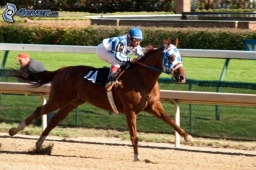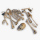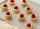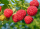# Animal species

Of 100 types of animals, 9/100 were discovered in ancient times, and 2/100 were discovered in the Middle Ages. Another 3/10
were discovered in the 1800s. What fraction of the 100 types of animals was discovered after the 1800s? Explain.

x =  0.59

### Step-by-step explanation:Did you find an error or inaccuracy? Feel free to write us. Thank you!Tips to related online calculators
Need help to calculate sum, simplify or multiply fractions? Try our fraction calculator.

## Related math problems and questions:

• The machineThe machine was in operation for 9 tenths (in a fraction) of eight hours of working time. How long has he been running?
• Human 3Human bones are 3/10 living tissues 9/20 minerals, and the rest water. What fraction of human bone is water?
• Simplest form of a fractionWhich one of the following fraction after reducing in simplest form is not equal to 3/2? a) 15/20 b) 12/8 c) 27/18 d) 6/4
• Evaluate mixed expressionsWhich of the following is equal to 4 and 2 over 3 divided by 3 and 1 over 2? A. 4 and 2 over 3 times 3 and 2 over 1 B. 14 over 3 times 2 over 7 C. 14 over 3 times 7 over 2 D. 42 over 3 times 2 over 31
• ShopperEva spent 1/4 in one store and 1/3 in another. What fraction is left?
• A textileA textile store sold a bolt of denim (what jeans are made out of). In one day, the following number of yards were purchased from the one bolt: 5 2/3, 7, 4 2/3, 8 5/8, 9 3/5, 10 ½, and 8. How many yards were sold?
• Fractions and mixed numerals(a) Convert the following mixed numbers to improper fractions. i. 3 5/8 ii. 7 7/6 (b) Convert the following improper fraction to a mixed number. i. 13/4 ii. 78/5 (c) Simplify these fractions to their lowest terms. i. 36/42 ii. 27/45 2. evaluate the follow
• MichaelMichael had a bar of chocolate. He ate 1/2 of it and gave away 1/3. What fraction had he left?
• Simplify 7Simplify. 7-1/3÷ 3-2/3 of 2+ 4-1/2÷ 2-1/4+ 1/2 solution and by step by step
• Guess a fractionTom was asked to guess a fraction. The sum of 1/2 the numerator and 1/3 of its denominator is 30. If Tom subtracts 36 from its denominator, the fraction becomes 1/3. What is the fraction that Tom was asked to guess? (Leave your answer in simplest form)
• There 14There are 250 people in a museum. 2/5 of the 250 people are girls 3/10 of the 250 people are boys The rest of the 250 people are adults Work out the number of adults in the museum.
• TartletsAfter a special event, a caterer examined the leftovers on the serving table. She saw 10/11 of a tartlet with apples, 2/3 of a tartlet with strawberries, and 10/11 of a tartlet with raspberries. How many leftover tartlets did the caterer have?
• Two numbers and its productThe product of two numbers are 2/3. If on of them is 1/10, what is the other?
• CarrieCarrie picked 2/5 of the raspberries from the garden, and Robin picked some too.  When they were finished, 1/3 of the raspberries still needed to be picked.  What fraction of the raspberries did Robin pick? Use pictures, numbers or words and write your fi
• FractionsSort fractions z1 = (6)/(11); z2 = (10)/(21); z3 = (19)/(22) by its size. Result write as three serial numbers 1,2,3.
• Length subtractingExpress in mm: 5 3/10 cm - 2/5 mm
• Simplify 3Simplify mixed numerals expression: 8 1/4- 3 2/5 - (2 1/3 - 1/4) Show your solution.